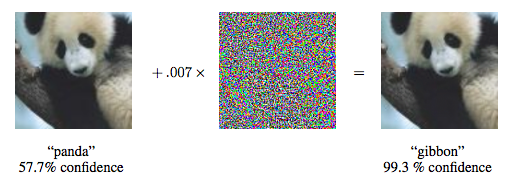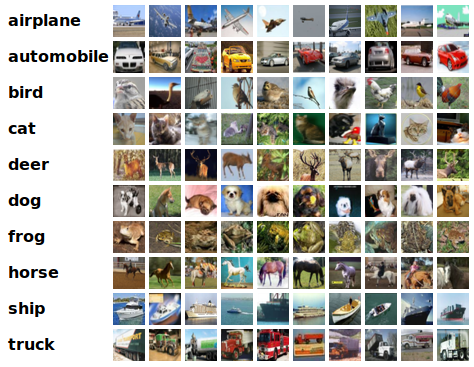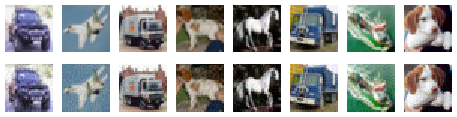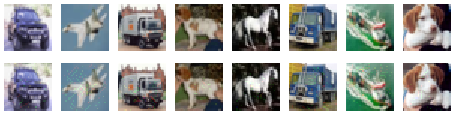# 深度学习的恶意样本实践`from os.path import abspathimport sysimport osimport tensorflow as tfsys.path.append(abspath('.'))import kerasimport numpy as npimport pickleimport matplotlib.pyplot as plt%matplotlib inlinefrom keras.datasets import cifar10from keras.models import load_modelfrom keras.utils import to_categoricalfrom art.classifiers import KerasClassifierfrom art.attacks.fast_gradient import FastGradientMethodfrom art.attacks.iterative_method import BasicIterativeMethodfrom art.attacks.saliency_map import SaliencyMapMethodfrom art.attacks import DeepFoolmy_model = load_model('cifar10_ResNet32v1_model.189.h5')(x_train, y_train), (x_test, y_test) = cifar10.load_data()x_train = x_train.astype('float32') / 255x_test = x_test.astype('float32') / 255x_train_mean = np.mean(x_train, axis=0)x_train -= x_train_meanx_test -= x_train_meanmin_ = np.min(x_train) max_ = np.max(x_train)`

`num_classes = 10y_train_vector = keras.utils.to_categorical(y_train, num_classes)y_test_vector = keras.utils.to_categorical(y_test, num_classes)scores = my_model.evaluate(x_test, y_test_vector, verbose=1)print('Test accuracy:', scores)`

`10000/10000 [==============================] - 4s 375us/stepTest accuracy: 0.9196`

`len_index = x_test.shapesum_success = 0for i in range(len_index): y_pred = my_model.predict(x_test[i].reshape(1,32,32,3)) if (np.argmax(y_pred) == y_test[i]):  sum_success  = 1print('Test accuracy:', sum_success/10000.0)`

`Test accuracy: 0.9196`

FGSM是一种比较简单的AE生成算法，它由来自谷歌大脑的三位研究者在文献【4】中提出，其中一位作者就是大名鼎鼎的 Goodfellow。Ian�Goodfellow因提出了生成对抗网络（GANs）而闻名，他被誉为“GANs之父”，甚至被认为是当前人工智能领域的顶级专家。他还是著名的花书《Deep Learning》的作者。文献【4】中的另外一个作者是Samy Bengio，他除了是深度学习领域的一位知名专家以外，还是新晋图灵奖得主Yoshua Bengio的兄弟。IBM ART工具箱中的FastGradientMethod函数实现了这个算法，下面我们就调用这个函数来对之前训练好的ResNet32网络发动AE攻击。

`x_test_1K, y_test_1K = x_test[:1000], y_test_vector[:1000]classifier = KerasClassifier((min_, max_), model=my_model)# Craft adversarial samples with FGSMepsilon = 0.03  # Maximum perturbationadv_fgsm_crafter = FastGradientMethod(classifier)x_test_adv_fgsm = adv_fgsm_crafter.generate(x=x_test_1K, eps=epsilon)scores_adv_test = my_model.evaluate(x_test_adv_fgsm, y_test_1K, verbose=1)print('Accuracy on Test AEs based on FGSM:', scores_adv_test)`

`1000/1000 [==============================] - 0s 309us/stepAccuracy on Test AEs based on FGSM: 0.187`

`fig=plt.figure(figsize=(8, 2))columns = 8rows = 2for i in range(1, 9): img = x_test[i 8].reshape(32,32,3)x_train_mean fig.add_subplot(rows, columns, i) plt.imshow(img) plt.axis('off') for i in range(1, 9): img_adv = x_test_adv_fgsm[i 8].reshape(32,32,3)x_train_mean fig.add_subplot(rows, columns, i 8) plt.imshow(img_adv) plt.axis('off')``for i in range(9, 17):� � y_pred = my_model.predict(x_test[i].reshape(1,32,32,3))� � print(np.argmax(y_pred), end = ' ')print("")for i in range(9, 17):� � y_pred = my_model.predict(x_test_adv_fgsm[i].reshape(1,32,32,3))� � print(np.argmax(y_pred), end = ' ')`

`1 0 9 5 7 9 8 5 9 3 1 4 6 1 6 3 `

`epsilon=0.015  # Maximum perturbationstepsize=0.005adv_igsm_crafter = BasicIterativeMethod(classifier, eps=epsilon, eps_step=stepsize)x_test_adv_igsm = adv_igsm_crafter.generate(x=x_test_1K)scores_adv_test = my_model.evaluate(x_test_adv_igsm, y_test_1K, verbose=1)print('Accuracy on Test AEs based on IGSM:', scores_adv_test)`

`1000/1000 [==============================] - 0s 317us/stepAccuracy on Test AEs based on IGSM: 0.063``for i in range(9, 17): y_pred = my_model.predict(x_test[i].reshape(1,32,32,3)) print(np.argmax(y_pred), end = ' ')print("")for i in range(9, 17): y_pred = my_model.predict(x_test_adv_igsm[i].reshape(1,32,32,3)) print(np.argmax(y_pred), end = ' ')`

`1 0 9 5 7 9 8 5 9 4 1 4 1 1 6 3 `

2016年，发表在CVPR上的文献【6】中提出了一种AE生成算法——DeepFool。IBM ART工具箱中的DeepFool函数实现了这个算法，其中需要注意的参数是max_iter 和epsilon，前者是生成算法的最大迭代次数，后者表示最大的扰动程度。

`# Craft adversarial samples with DeepFooladv_df_crafter = DeepFool(classifier, max_iter=50, epsilon=0.01)x_test_adv_df = adv_df_crafter.generate(x_test_1K)scores_adv_test = my_model.evaluate(x_test_adv_df, y_test_1K, verbose=1)print('Accuracy on Test AEs based on DeepFool:', scores_adv_test)`

`1000/1000 [==============================] - 0s 297us/stepAccuracy on Test AEs based on DeepFool: 0.325``for i in range(9, 17): y_pred = my_model.predict(x_test[i].reshape(1,32,32,3)) print(np.argmax(y_pred), end = ' ')print("")for i in range(9, 17): y_pred = my_model.predict(x_test_adv_df[i].reshape(1,32,32,3)) print(np.argmax(y_pred), end = ' ')`

`1 0 9 5 7 9 8 5 9 4 1 4 7 8 6 7 `

JSMA是由Nicolas Papernot等在2016年的Euro S&P上提出的一种类型的AE生成算法，参见文献【5】。与前面介绍的几种算法不同，JSMA是一种Targeted的攻击算法。也就是说，它不仅可以误导神经网络输出错误的分类结果，还可以预设一个目标，让神经网络输出指定的（错误）分类结果。因此，我们需要先给出预设的分类目标。这里采取的方法为，总是令神经网络输出原本的正确标签的下一个作为目标标签，例如正确的标签本应该是8，则AE会误导神经网络输出9，如果正确的标签本应该是9，则AE会误导神经网络输出0... ... 下面的代码用于生成预设之标签：

`test_len = y_test.shapey_test_t = np.zeros(y_test.shape)for i in range(test_len): y_test_t[i] = (y_test[i]1)%num_classesy_test_t_vector = keras.utils.to_categorical(y_test_t, num_classes)y_test_target = y_test_t_vector[:1000]`

IBM ART工具箱中的SaliencyMapMethod函数实现了JSMA算法，下面的代码就调用这个函数来对之前训练好的ResNet32网络发动AE攻击。

`# Craft adversarial samples with JSMAadv_jsma_crafter = SaliencyMapMethod(classifier, theta=0.1)x_test_adv_jsma = adv_jsma_crafter.generate(x_test_1K, y = y_test_target)scores_adv_test = my_model.evaluate(x_test_adv_jsma, y_test_1K, verbose=1)print('Accuracy on Test AEs based on JSMA:', scores_adv_test)``for i in range(9, 17): y_pred = my_model.predict(x_test[i].reshape(1,32,32,3)) print(np.argmax(y_pred), end = ' ')print("")for i in range(9, 17): y_pred = my_model.predict(x_test_adv_jsma[i].reshape(1,32,32,3)) print(np.argmax(y_pred), end = ' ')`

`1 0 9 5 7 9 8 5 2 1 0 6 8 0 9 6 `

【4】Alexey Kurakin, Ian Goodfellow, Samy Bengio, Adversarial examples in the physical world,�https://arxiv.org/abs/1607.02533

【5】Nicolas Papernot, Patrick McDaniel, Somesh Jha, Matt Fredrikson, Z. Berkay Celik, Ananthram Swami,�The Limitations of Deep Learning in Adversarial Settings,�IEEE European Symposium on Security & Privacy, 2016

【6】Seyed-Mohsen Moosavi-Dezfooli, Alhussein Fawzi, Pascal Frossard, DeepFool: a simple and accurate method to fool deep neural networks, CVPR, 2016

（全文完）